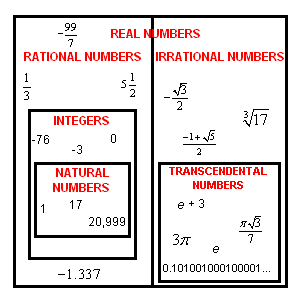# Irrational Numbers

An irrational number is a real number that cannot be expressed in the form $\frac{a}{b}$ , when $a$ and $b$ are integers ( $b\ne 0$ ).  In decimal form, it never terminates (ends) or repeats.

The ancient Greeks discovered that not all numbers are rational ; there are equations that cannot be solved using ratios of integers.

The first such equation to be studied was $2={x}^{2}$ . What number times itself equals $2$ ?

$\sqrt{2}$ is about $1.414$ , because ${1.414}^{2}=1.999396$ , which is close to $2$ . But you'll never hit exactly by squaring a fraction (or terminating decimal ). The square root of $2$ is an irrational number, meaning its decimal equivalent goes on forever, with no repeating pattern:

$\sqrt{2}=1.41421356237309...$

Historical Note:

According to legend, the ancient Greek mathematician who proved that $\sqrt{2}$ could NOT be written as a ratio of integers $\frac{p}{q}$ made his colleagues so angry that they threw him off a boat and drowned him!

Other famous irrational numbers are the golden ratio , a number with great importance to biology:

$\frac{-1\text{\hspace{0.17em}}+\text{\hspace{0.17em}}\sqrt{5}}{2}=0.61803398874989...$

$\pi$ (pi) , the ratio of the circumference of a circle to its diameter:

$\pi =3.14159265358979...$

$e=2.71828182845904...$

Irrational numbers can be further subdivided into algebraic numbers, which are the solutions of some polynomial equation (like $\sqrt{2}$ and the golden ratio), and transcendental numbers, which are not the solutions of any polynomial equation. $\pi$ and $e$ are both transcendental.

The Venn diagram below shows the relationships of the various sets of numbers.